# Law Of Gravitation PPT For All The Students | With Modern Animations and Infographics | Jay Butani

Android Developer à Par Infotech
1 Dec 2019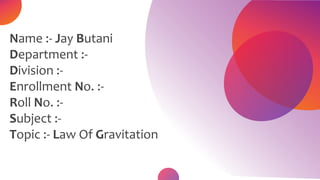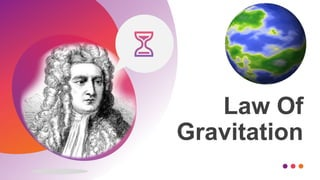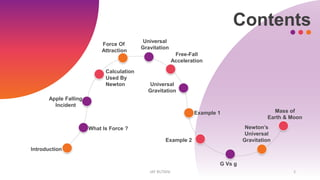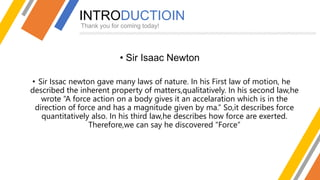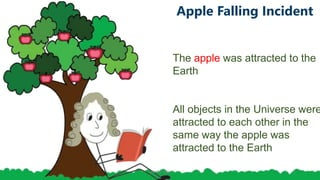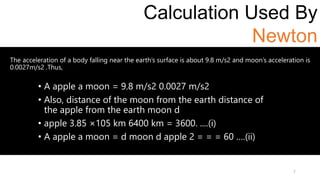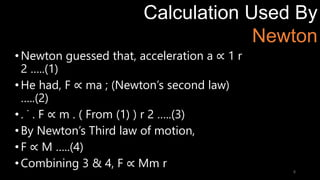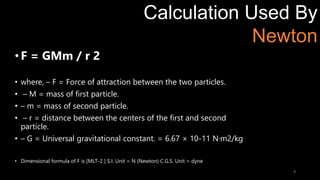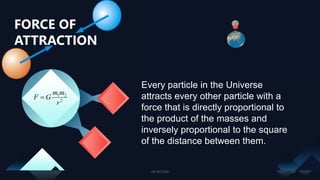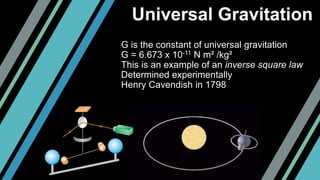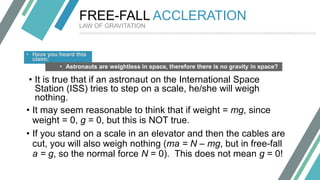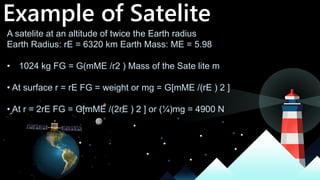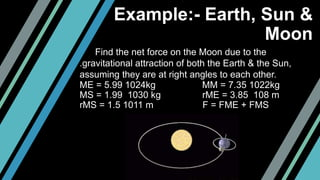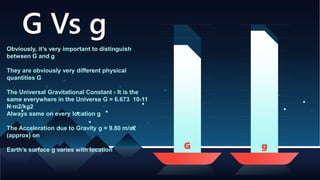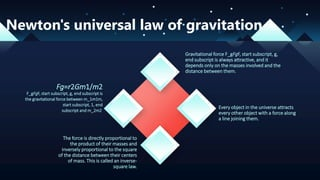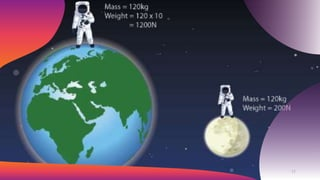1 sur 18

### Law Of Gravitation PPT For All The Students | With Modern Animations and Infographics | Jay Butani

• 1. Name :- Jay Butani Department :- Division :- Enrollment No. :- Roll No. :- Subject :- Topic :- Law Of Gravitation
• 2. Law Of Gravitation
• 3. Apple Falling Incident Universal Gravitation Example 1 Force Of Attraction Free-Fall Acceleration Contents JAY BUTANI 3 Universal Gravitation Example 2 G Vs g Newton’s Universal Gravitation Mass of Earth & Moon Introduction What Is Force ? Calculation Used By Newton
• 4. INTRODUCTIOIN Thank you for coming today! • Sir Isaac Newton • Sir Issac newton gave many laws of nature. In his First law of motion, he described the inherent property of matters,qualitatively. In his second law,he wrote “A force action on a body gives it an accelaration which is in the direction of force and has a magnitude given by ma.” So,it describes force quantitatively also. In his third law,he describes how force are exerted. Therefore,we can say he discovered “Force”
• 5. The force is an external effort(cause) in the form of a push or pull which either changes or tends to change the state of rest or the uniform motion of a body along a straight line. They are classified into two categories:- (i) Contact Force. - Frictional force, normal reaction, tensile force etc. (ii) (ii) Non-Contact Force. - electric, magnetic, gravitational force. What is FORCE ?
• 6. Apple Falling Incident aftr The apple was attracted to the Earth All objects in the Universe were attracted to each other in the same way the apple was attracted to the Earth JAY BUTANI 6
• 7. 7 Calculation Used By Newton The acceleration of a body falling near the earth’s surface is about 9.8 m/s2 and moon’s acceleration is 0.0027m/s2 .Thus, • A apple a moon = 9.8 m/s2 0.0027 m/s2 • Also, distance of the moon from the earth distance of the apple from the earth moon d • apple 3.85 ×105 km 6400 km = 3600. ....(i) • A apple a moon = d moon d apple 2 = = = 60 ….(ii)
• 8. 8 Calculation Used By Newton •Newton guessed that, acceleration a ∝ 1 r 2 …..(1) •He had, F ∝ ma ; (Newton’s second law) …..(2) •. ˙ . F ∝ m . ( From (1) ) r 2 …..(3) •By Newton’s Third law of motion, •F ∝ M …..(4) •Combining 3 & 4, F ∝ Mm r
• 9. 9 Calculation Used By Newton •F = GMm / r 2 • where, – F = Force of attraction between the two particles. • – M = mass of first particle. • – m = mass of second particle. • – r = distance between the centers of the first and second particle. • – G = Universal gravitational constant. = 6.67 × 10-11 N·m2/kg • Dimensional formula of F is [MLT-2 ] S.I. Unit = N (Newton) C.G.S. Unit = dyne
• 10. 2 21 r mm GF  FORCE OF ATTRACTION Every particle in the Universe attracts every other particle with a force that is directly proportional to the product of the masses and inversely proportional to the square of the distance between them. JAY BUTANI 10
• 11. Universal Gravitation G is the constant of universal gravitation G = 6.673 x 10-11 N m² /kg² This is an example of an inverse square law Determined experimentally Henry Cavendish in 1798
• 12. FREE-FALL ACCLERATION • Have you heard this claim: • Astronauts are weightless in space, therefore there is no gravity in space? • It is true that if an astronaut on the International Space Station (ISS) tries to step on a scale, he/she will weigh nothing. • It may seem reasonable to think that if weight = mg, since weight = 0, g = 0, but this is NOT true. • If you stand on a scale in an elevator and then the cables are cut, you will also weigh nothing (ma = N – mg, but in free-fall a = g, so the normal force N = 0). This does not mean g = 0! LAW OF GRAVITATION
• 13. Example of Satelite A satelite at an altitude of twice the Earth radius Earth Radius: rE = 6320 km Earth Mass: ME = 5.98 • 1024 kg FG = G(mME /r2 ) Mass of the Sate lite m • At surface r = rE FG = weight or mg = G[mME /(rE ) 2 ] • At r = 2rE FG = G[mME /(2rE ) 2 ] or (¼)mg = 4900 N
• 14. Example:- Earth, Sun & Moon Find the net force on the Moon due to the .gravitational attraction of both the Earth & the Sun, assuming they are at right angles to each other. ME = 5.99 1024kg MM = 7.35 1022kg MS = 1.99 1030 kg rME = 3.85 108 m rMS = 1.5 1011 m F = FME + FMS
• 15. G Vs g G g Obviously, it’s very important to distinguish between G and g They are obviously very different physical quantities G The Universal Gravitational Constant › It is the same everywhere in the Universe G = 6.673 10-11 N∙m2/kg2 Always same on every location g The Acceleration due to Gravity g = 9.80 m/s2 (approx) on Earth’s surface g varies with location
• 16. Gravitational force F_gFg​F, start subscript, g, end subscript is always attractive, and it depends only on the masses involved and the distance between them. Every object in the universe attracts every other object with a force along a line joining them. Fg​=r2Gm1/​m2 F_gFg​F, start subscript, g, end subscript is the gravitational force between m_1m1​m, start subscript, 1, end subscript and m_2m2 Newton's universal law of gravitation The force is directly proportional to the product of their masses and inversely proportional to the square of the distance between their centers of mass. This is called an inverse- square law.
• 17. JAY BUTANI 17
• 18. That’s all. Thank you! 